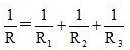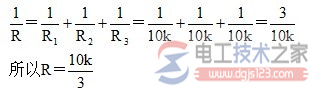# 并联电阻计算公式_多电阻并联的计算方法

## 并联电阻的计算公式

1、计算公式2、计算方法R=R1R2/(R1+R2)=

R1=R2R/(R2-R)=

R2=R1R/(R1-R)=

1/R=1/R1+1/R2+-------+1/Rn.

R=1/(1/R1+1/R2+...+1/Rn)

U/R总=U/R1+U/R2+...+U/Rn

n个电阻的并联，并联后的电阻   R总=1/(1/R1+1/R2+1/R3+……+1/Rn)   把各个电阻的阻值代入   就可以求出来总电阻了

1/R总=1/R1+1/R2+1/R3+......+1/Rn

1、如果三个一样的电阻并联的话，不用算的，直接除以3，即10k/3欧。

2、详细如下图：(三个电阻不一样也是这个公式) 。【并联电阻计算公式_多电阻并联的计算方法】

I总=I1+I2+......+In

U总=U1=U2=Un

1/R总=1/R1+1/R2+1/R3+......+1/Rn

`相关文章`